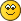Options

# Hypervector Analysis of the Voynich Manuscript

• #### Index

• Hypervector Analysis of the Voynich Manuscript
• ### RE: Hypervector Analysis of the Voynich Manuscript

ReneZ > 01-09-2020, 05:59 AM

Well, of course, PCA can give any number of components, up to a limit N where N is the smallest of:
- the length of the vectors in terms of number of coordinates (here 10,000)
- the number of vectors (here of the order of 60 if I remember correctly)

However, Darren is only showing the first two. In an earlier experiment I did, I plotted PCA 2 vs.1, 3 vs. 1 and 4 vs. 1
(see You are not allowed to view links. Register or Login to view.  about halfway down the page)

One can indeed compute how much of the variance is 'contained' in each component, and this number goes down for each higher component.
It is theoretically possible that a number of the expected dimensions (N) have a very small or even zero variance, but that is only the case if the vectors are linearly dependent. In the present case, it is not at all to be expected that components 3, 4, 5 are very small, and as I mentioned before, there is a clear indication that they are there.

The PCA directions are computed from the data, and if one adds one vector to the set, the PCA directions will change.

I will try to show where I see this effect, later today.

(I am aware that you know, but: ) one can also compute the total variance that is 'left' after removing the first two principal components, and one has a clear indication ''how much' we are not yet seeing in the plot.
• ### RE: Hypervector Analysis of the Voynich Manuscript

ReneZ > 01-09-2020, 08:28 AM

Here are three 'cuts' of PCA plots that have been shown in this thread. One can see that the points are moving, which means that the principal axes are rotating. This is the effect of the higher components which are not visible in each plot due to the projection of 50+ dimensions to only 2. Unfortunately, I am not entirely sure which other changes were made apart from adding more Voynich transliterations. (I believe that Romani was added at one point as well).

First and second plots:One can see how the points on the right have moved due to the new projection, and how the Voynich point, which was originally vertically between Andi, Ossetic and Agul, has now moved outside. This is the result of separations in higher dimensions.

In the third plot theree are three versions of Voynichese:and now the Voynich points have also moved 'beyond'' Adyghe.
One can observe the significant rotations also from the relative positions of Mandarin and Korean, or Abaza and Mongolian, among others.
• ### RE: Hypervector Analysis of the Voynich Manuscript

dvallis > 06-09-2020, 06:58 PM

The best analogy I have for the PCA plot is shining a flashlight through a snow globe and looking at the shadows on a wall. Example of 3D to 2D dimensional reduction. If points are close together in 3D they will not move much in relation to each other in 2D as the globe is rotated. Same should apply for 10KD to 2D.

In our case the apply( ) and prcomp( ) functions are doing all the work. I haven't been able to figure out how to control rotation using them so far.

I recently acquired a VR headset. I'm wondering how to do PCA in 3D, i.e. decompose 10KD to 3D. If we can come up with an algorithm I'll code it it CUDA for real time 3D viewing and rotation in VR. Think Minority Report for language comparison.

v = v[,which(apply(v,2,var)!=0)]
v.pca <- prcomp(v,scale. = TRUE, center = TRUE)
fviz_pca_biplot(v.pca, invisible = “var”,title=”Hypervector Language Comparison PCA Plot”,repel=TRUE,axes.linetype = “blank”)
• ### RE: Hypervector Analysis of the Voynich Manuscript

dvallis > 06-09-2020, 07:15 PM

Ah Ha! PCA in 3D. Stay tuned for results.

You are not allowed to view links. Register or Login to view.
• ### RE: Hypervector Analysis of the Voynich Manuscript

dvallis > 07-09-2020, 04:32 AM

Well, this turned out frigging amazing don't you think? Much better than the previous 2D "snow globe & flashlight" view.Here's the process in R-Studio

#Setup
instal.packages("rgl")

#For RGL
library(rgl)

#Remove zero variance columns
v = v[,which(apply(v,2,var)!=0)]

#Generate PCA
v.pca <- prcomp(v,scale. = TRUE, center = TRUE)
data=as.data.frame(v.pca\$x)

#Plot it
plot3d(data[,1:3], col=c(1:4), size=10, type='p')
text3d(data[,1]+1, data[,2]+1, data[,3]+1,texts=c(rownames(data)), cex= 0.7, pos=3)

#Rotate it
for (i in 1:360) {
rgl.viewpoint(theta=i, phi=30, fov=60, zoom = 0.4)
Sys.sleep(.05)}

#Save 360 PNG images
dir.create("an-gif")
for (i in 1:360) {
rgl.viewpoint(theta=i, phi=30, fov=60, zoom = 0.5)
rgl.snapshot(filename=paste("an-gif/frame-",
sprintf("%03d", i), ".png", sep=""))}

Then convert 360 PNGs to a motion GIF with You are not allowed to view links. Register or Login to view..

Pretty happy with these results. Very cool.
• ### RE: Hypervector Analysis of the Voynich Manuscript

dvallis > 15-09-2020, 03:09 AM

Just for fun, I threw in Klingon• ### RE: Hypervector Analysis of the Voynich Manuscript

-JKP- > 15-09-2020, 08:53 AM